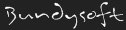L3DT documentationLarge 3D terrain generator

# vector3f

## Description

`vector3f` is structure used to describe a 3-dimensional vector in floating-point precision. This data type is more precise than vector3c but is less compact. Therefore, `vector3f` is used for several calculation settings (for greater accuracy), but is not used in vector map data (for space reasons).

Vectors may or may not be normalised.

## Definition

In Zeolite_defines.h:

```typedef struct {
float x;
float y;
float z;
} vector3f;```

## Member data

x float The vector component in the x-axis.
y float The vector component in the y-axis.
z float The vector component in the z-axis.

## Type identifiers

 VarID VarID_vector3f N/A (vector3f is not a valid map type.)

Although the vector components are named x, y and z, these members may be used to describe any 3-dimensional vector. As an example, the light-mapping algorithm in L3DT uses a `vector3f` struct to describe light absorption, and the three members represent the absorption half-depths of red, green and blue light.